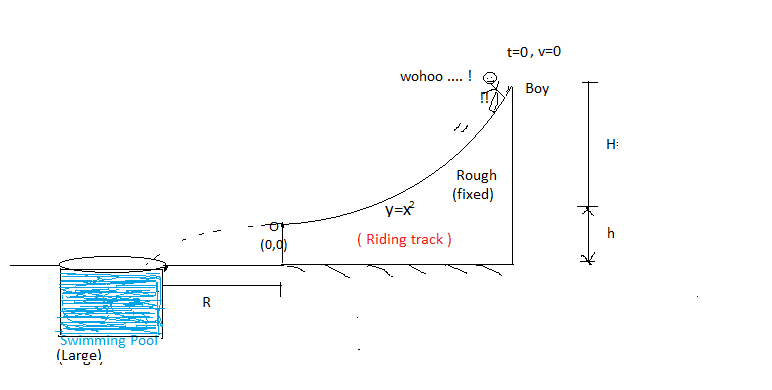# Fearless Boy says ''Let's do some different"An fearless boy , want to do stunt . He reach on the top of rough fixed riding track which has coefficient of friction $\mu$ and released his body from rest, so that it leaves the Parabolic($y=x^2$) riding track at the origin, and then finally wish to fall in swimming pool, so that he doesn't hurt.

If value of $R$ is such that , boy will Just fall in the swimming pool. If it is expressed as :

${ R=\sqrt { { me }^{ -\cfrac { \pi }{ n } }-\cfrac { p }{ q } } \\ }$

here $m,n,p,q$ are positive integers and with $p,q$ coprime.

Find $m+n+p+q$

Details and assumptions

• $\displaystyle{{ \mu =0.5\\ g=10m/{ s }^{ 2 }\\ H=0.75m\quad \\ h=0.5m }}$

• e is Euler's constant.

×